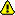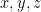[Documentation] [TitleIndex] [WordIndex]The sensor element has been implemented in the URDF Dom but has never really been used in application. This is a project that was dropped anyone is encouraged to pick it up and extend it to sensor hardware applications. Please contribute!

## <sensor> element

The sensor element describes basic properties of a visual sensor (i.e. camera / ray sensor).

Here is an example of a camera sensor element:

```   1  <sensor name="my_camera_sensor" update_rate="20">
3    <origin xyz="0 0 0" rpy="0 0 0"/>
4    <camera>
5      <image width="640" height="480" hfov="1.5708" format="RGB8" near="0.01" far="50.0"/>
6    </camera>
7  </sensor>
```

And below is an example of a laser scan (ray) sensor element:

```   1  <sensor name="my_ray_sensor" update_rate="20">
3    <origin xyz="0 0 0" rpy="0 0 0"/>
4    <ray>
5      <horizontal samples="100" resolution="1" min_angle="-1.5708" max_angle="1.5708"/>
6      <vertical samples="1" resolution="1" min_angle="0" max_angle="0"/>
7    </ray>
8  </sensor>
```

## Attributes

• name (required) (string)

• The name of the link itself
• update_rate (optional) (float) (Hz)

• The frequency at which the sensor data is generated. If left unspecified, the sensor will generate data every cycle.

## Elements

• <parent> (required)

• The name of the link this sensor is attached to.

<origin> (optional: defaults to identity if not specified)

• This is the pose of the sensor optical frame, relative to the sensor parent reference frame. The sensor optical frame adopts the conventions of z-forward, x-right and y-down.
• xyz (optional: defaults to zero vector)

• Represents theoffset.

• rpy (optional: defaults to identity if not specified)

• Represents the fixed axis roll, pitch and yaw angles in radians.
• <camera> (optional)

• <image> (required)

• width (required) (unsigned int) (pixels)

• Width of the camera in pixels.
• height (required) (unsigned int) (pixels)

• Height of the camera in pixels.
• format (required) (string)

• Horizontal field of view of the camera
• near (required) (float) (m)

• Near clip distance of the camera in meters.
• far (required) (float) (m)

• Far clip distance of the camera in meters. This needs to be greater or equal to near clip.
• <ray> (optional)

• <horizontal> (optional)

• samples (optional: default 1) (unsigned int)

• The number of simulated rays to generate per complete laser sweep cycle.
• resolution (optional: default 1) (float)

• This number is multiplied by samples to determine the number of range data points returned. If resolution is less than one, range data is interpolated. If resolution is greater than one, range data is averaged.
• min_angle (optional: default 0) (float) (radians)

• max_angle (optional: default 0) (float) (radians)

• Must be greater or equal to min_angle
• <vertical> (optional)

• samples (optional: default 1) (unsigned int)

• The number of simulated rays to generate per complete laser sweep cycle.
• resolution (optional: default 1) (float)

• This number is multiplied by samples to determine the number of range data points returned. If resolution is less than one, range data is interpolated. If resolution is greater than one, range data is averaged.
• min_angle (optional: default 0) (float) (radians)

• max_angle (optional: default 0) (float) (radians)

• Must be greater or equal to min_angle

• In simulation, large sensors will slow down overall performance. Depending on update rates required, it is recommended to keep the camera or ray resolution and update rates as low as possible.

2020-01-18 13:18